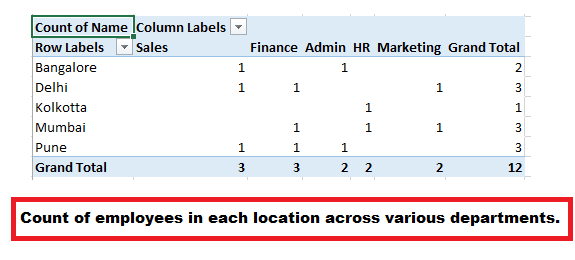# Excel Macros Series 3: Create Pivot Charts to get employee count across locations via department.

In our below example we have a data of employee data dashboard across locations.Here instead of using the conventional method for creating a Pivot Table, we use Excel VBA macro instead to represent our required dashboard.

This code can be used in combination with our previous excel macro series example.

Eg: Extract active employee data onto a separate sheet and then create a pivot table based on the results.

Also we have created a code which will delete the Pivot charts in the workbook at the click of a command button.```
Sub Create_PivotTable()

Dim pc As PivotCache
Dim PT As PivotTable
Dim pf As PivotField

'wsdata is the name of the worksheet
If ThisWorkbook.PivotCaches.Count = 0 Then
Set pc = ThisWorkbook.PivotCaches.Create( _
SourceType:=xlDatabase, _
SourceData:=wsdata.Name & "!" & wsdata.Range("A1").CurrentRegion.Address, _
Version:=xlPivotTableVersion15)
Else
Set pc = ThisWorkbook.PivotCaches(1)
End If

Range("A3").Select

Set PT = pc.CreatePivotTable( _
Tabledestination:=ActiveCell, _
TableName:="dataPivot")

' Row parameter for pivot table
Set pf = PT.PivotFields("Location")
pf.Orientation = xlRowField

' Column parameter for pivot table

Set pf = PT.PivotFields("Department")
pf.Orientation = xlColumnField

' Sum /Average /COunt etc parameter for pivot table
Set pf = PT.PivotFields("Name")
pf.Orientation = xlDataField
' you can use functions like xlsum, xlaverage
pf.Function = xlCount

End Sub

Sub DeleteAllPivotTablesInWorkbook()
Dim WS as Worksheet
On Error Resume Next
For Each WS In ActiveWorkbook.Worksheets
For Each PT In WS.PivotTables